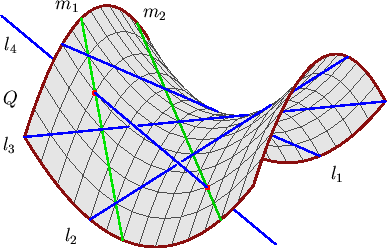Next: 4.ii. The Special Schubert Calculus

# 4.i. The Schubert Calculus of Lines

The first non-trivial instance of the Schubert calculus is the question posed at the beginning of Section 3.i.

Question 4.1   How many lines in space meet four general lines l1, l2, l3, and l4?

Three pairwise skew lines l1, l2, and l3 lie on a unique smooth quadric surface Q. There are two families of lines that foliate Q--one family includes l1, l2, and l3 and the other consists of the lines meeting each of l1, l2, and l3. The fourth line l4 meets Q in two points, and each of these points determines a line in the second family. Thus there are 2 lines m1 and m2 in space that meet general lines l1, l2, l3, and l4. Figure 10 shows this configuration.Figure 10: The two lines meeting four general lines in space.

Figure 10 also shows how the two lines can be real--if l4 meets Q in two real points. (The two lines are complex when l4 meets Q in two complex conjugate points.)

The classical Schubert calculus is a vast generalization of this problem of four lines. In the 1980's Speiser suggested to Fulton that the classical Schubert calculus may be a good testing ground for Question 3.1. This was also considered by Chiavacci and Escamilla-Castillo [CE-C]. We will discuss increasingly more general versions of the Schubert calculus, and the status of Question 3.1 for each.

Consider first more general problems involving lines. The space of lines in Pn is a smooth projective variety of dimension 2n - 2 called the Grassmannian of lines in Pn. The set of lines meeting a linear subspace L of dimension n-1-l has codimension l in the Grassmannian. Thus given general linear subspaces L1, L2, ..., Ls, of Pn with the dimension of Li equal to n-1-li, where we have l1 + l2 + ... + ls = 2n - 2, we expect (and there are indeed) finitely many lines in Pn meeting each linear subspace L1, L2, ..., Ls. Schubert [Sch3] discovered algorithms for computing this number d(l1,l2,...,Ls) of lines. For example, if each li is 1, so that s = 2n - 2, then this number is the nth Catalan number§(4.1)

Thus there are Cn lines meeting each of 2n - 2 codimension 2 planes L1, L2, ..., L2n-2 in Pn.

These enumerative problems of lines in Pn meeting general linear subspaces furnished the first infinite family of non-trivial enumerative problems known to be fully real.

Theorem 4.2 ([So1, Theorem C)   Given positive integers l1, l2, ..., ls with l1 + l2 + ... + ls = 2n - 2, there exist real linear subspaces L1, L2, ..., Ls, of Pn with dimLi = n-1-li such that there are exactly d(l1,l2,...,Ls) complex lines meeting each subspace Li, and each of these lines is real.

§This indexing of the Catalan numbers is shifted from that of some other authors.Next: 4.ii. The Special Schubert Calculus